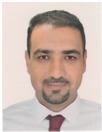###### MUTAH University##### Courses
Course Name Academic Year Description Course Name Academic Year Description Electric Circuits 1 Second Year Fundamental principles of circuit theory commonly used in engineering research and scientific applications. Techniques and principles of electrical circuit analysis, including basic concepts such as voltage, current, resistance, impedance, Ohm’s and Kirchhoff’s law; basic electric circuit analysis techniques, resistive circuits, transient and steady-state response of RLC circuits; circuits with AC and sinusoidal sources, Power Calculations Electric Circuits 2 Second Year General circuit analysis in Phasor domain. Three phase circuits and power calculations. Magnetic coupling and ideal transformer. One and two port networks transfer function and complex frequency. Resonant circuits filters types, analysis, and design, applications of Laplace and Fourier transforms in circuits. Electromagnetics Third Year Electrostatic fields and steady Magnetic fields laws and theories, Forces, materials, Resistances, capacitance and inductance of different geometries. Laplace's and Poisson's Equations. Introduction ,Static electric field; static electric field in dielectrics, electric current; the static magnetic field due to electric current; magnetization and magnetic fields; Ampare's Law, Magnetic Flux and Guass's Law; Forces, materials, Resistances, capacitance and inductance of different geometries. Laplace's and Poisson's Equations. Time Signals and Systems Second Year Complex numbers, Time and frequency domain representations and analysis of signals and systems. Convolving and linear input-output systems in continuous and discrete time. Fourier transforms .Fourier series for continuous and discrete time-signals, Laplace and Z-transforms Fields and Waves Fourth Year Time varying fields; Faraday's and Lenz's laws; transformer and motional electromotive force; displacement current;; Maxwell's equations; wave equations; time-harmonic fields; complex phasors; scalar and vector potential functions ;EM Waves and generation experiments; plane waves propagation in vacuum; plane waves in dielectrics and conductors; EM waves polarizations; skin effect; electromagnetic energy and power; Poynting's theorem; reflection and refraction of plane waves at dielectric interfaces; Snell's laws; Fresnel formulas; critical angle; total internal reflection; total transmission; Brewster's angle; standing waves; transmission line theory; TEM waves; transmission line parameters; lossy and lossless lines; matching of transmission lines to their loads using Smith Chart. Antennas and Waves Propagation Fifth Year Antenna principle of operation, radiation  mechanism, types  of  antennas and theirs
Parameters, dipoles, wire-antennas, microstrip patch antennas, arrays, and models
of propagation.
Microwave Engineering Fourth Year Review of Maxwell's Equations, types of transmission lines and modes of propagations wave impedance and characteristic impedances, Microwave waveguides,. Resonant circuits, losses and Q-factors, Microwave Networks parameters. Microwave passive devices: couplers, T, Magic T, Isolator, Circulator, , Antennas and Microwaves Lab Fifth Year Design and simulate RF and Microwave circuits and antennas. Students use CST Microwave Studio to simulate, verify, and optimize their design. Students learn how to measure radiation patterns. Numerical Methods and Data Analysis Second Year Error Analysis: Approximations and Round-Off Errors. Truncation Errors and the Taylor Series. Roots of Equations: Bracketing Methods. Open Methods. Roots of Polynomials Linear Algebraic Equations: Gauss Elimination.LU Decomposition and Matrix Inversion .Special Matrices and Gauss-Seidel.  Curve Fitting: Least-Squares Regression. Interpolation. Numerical Differentiation and Integration: Newton-Cotes Integration Formulas. Integration of Equations. Numerical Differentiation. Ordinary Differential Equations: Runge-Kutta Methods.
Optical Communications Fifth Year Introduction theory of transmission on optical fibers, sources, lasers and LEDs, detectors PIN and APD . Transmitters and receivers and the effect of noise. Optical Networks. Optical Link budget analysis. WDM. Digital Communications and Communications Systems LAB Fifth Year Sampling, Quantization and coding. Quantization errors. PCM and demodulation. ASK,FSK,&PSK. The relation between channel capacity, probability of error and signal to noise ratio and band width. Analog Communications LAB Fourth Year Measurements in the essential communication circuits. Modulators and demodulators super heterodyne receivers. Single sideband generation and demodulation. Electric Circuits LAB Second Year Experiments in DC circuits; circuits theorems; DC responses of RL, RC, and RLC; AC waveforms characteristics; three phase systems and power measurements; filter circuits Electronics LAB Third Year Measurement of the diode characteristics Applications on diode circuits, Transistor characteristics bipolar and FETs. Amplifiers, oscillators, Timers